## Definitions of the Determinant

The determinant function can be defined by essentially two different methods. The advantage of the first definition—one which uses permutations—is that it provides an actual formula for det A, a fact of theoretical importance. The disadvantage is that, quite frankly, no one actually computes a determinant by this method.

Method 1 for defining the determinant. If n is a positive integer, then a permutation of the set S = {1, 2, …, n} is defined to be a bijective function—that is, a one‐to‐one correspondence—σ, from S to S. For example, let S = {1, 2, 3} and define a permutation σ of S as follows: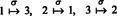Since σ(1) = 3, σ(2) = 1, and σ(3) = 2, the permutation σ maps the elements 1, 2, 3 into 3, 1, 2. Intuitively, then, a permutation of the set S = {1, 2, …, n} provides a rearrangement of the numbers 1, 2, …, n. Another permutation, σ′, of the set S is defined as follows: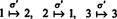This permutation maps the elements 1, 2, 3 into 2, 1, 3, respectively. This result is written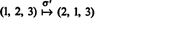Example 1: In all, there are six possible permutations of the 3‐element set S = {1, 2, 3}: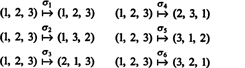In general, for the set S = {1, 2, …, n}, there are n! ( n factorial) possible permutations.

To transpose two adjacent elements simply means to interchange them; for example, the transposition (or inversion) of the pair 2, 3 is the pair 3, 2. Every permutation can be obtained by a sequence of transpositions. For example, consider the permutation σ 5 of S = {1, 2, 3} defined in Example 1 above. The result of this permutation can be achieved by two successive transpositions of the original set:Three transpositions are needed to give the permutation σ 6 of Example 1:The number of transpositions needed to recover a given permutation is not unique. For example, you could always intersperse two successive transpositions, the second one of which simply undoes the first. However, what is unique is whether the number of transpositions is even or odd. If the number of transpositions that define a permutation is even, then the permutation is said to be even, and its sign is +1. If the number of transpositions that define a permutation is odd, then the permutation is said to be odd, and its sign is −1. The notation is as follows:Note that sgn σ can be defined as (−1) t , where t is the number of transpositions that give σ.

Example 2: Determine the sign of the following permutation of the set S = {1, 2, 3, 4}:The “brute‐force” method is to explicitly determine the number of transpositions: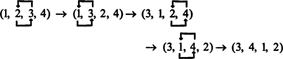Since σ can be achieved by 4 successive transpositions, σ is even, so its sign is +1.

A faster method proceeds as follows: Determine how many pairs within the permutation have the property that a larger number precedes a smaller one. For example, in the permutation (3, 4, 1, 2) there are four such pairs: 3 precedes 1, 3 precedes 2, 4 precedes 1, and 4 precedes 2. The fact that the number of such pairs is even means the permutation itself is even, and its sign is +1. [Note: The number of pairs of elements that have the property that a larger number precedes a smaller one is the minimum number of transpositions that define the permutation. For example, since this number is four for the permutation (3, 4, 1, 2), at least four transpositions are needed to convert (1, 2, 3, 4) into (3, 4, 1, 2); the specific sequence of these four transpositions is shown above.]

For every integer n ≥ 2, the total number of permutations, n!, of the set S = {1, 2, …, n} is even. Exactly half of these permutations are even; the other half are odd.

Example 3: For the 6 = 3! permutations of the set S = {1, 2, 3} given in Example 1, verify that the three permutations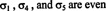and, therefore, each has sign +1, while the other three permutations,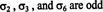and each has sign −1.

Now that the concepts of a permutation and its sign have been defined, the definition of the determinant of a matrix can be given. Let A = [ a ij ] be an n by n matrix, and let S n denote the collection of all permutations of the set S = {1, 2, …, n}. The determinant of A is defined to be the following sum:Example 4: Use definition (*) to derive an expression for the determinant of the general 2 by 2 matrixSince n = 2, there are 2! = 2 permutations of the set {1, 2}, namely,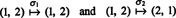The identity permutation, σ 1, is (always) even, so sgn σ 1 = +1, and the permutation σ 2 is odd, so sgn σ 2 = −1. Therefore, the sum (*) becomes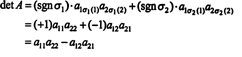This formula is one you should memorize: To obtain the determinant of a 2 by 2 matrix, subtract the product of the offdiagonal entries from the product of the diagonal entries: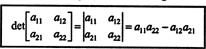To illustrate,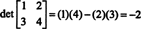Example 5: Use definition (*) to derive an expression for the determinant of the general 3 by 3 matrix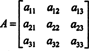Since n = 3, there are 3! = 6 permutations of {1, 2, 3}, and, therefore, six terms in the sum (*):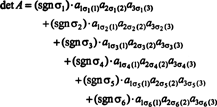Using the notation for these permutations given in Example 1, as well as the evaluation of their signs in Example 3, the sum above becomes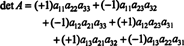or, more simply,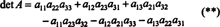As you can see, there is quite a bit of work involved in computing a determinant of an n by n matrix directly from definition (*), particularly for large n. In applying the definition to evaluate the determinant of a 7 by 7 matrix, for example, the sum (*) would contain more than five thousand terms. This is why no one ever actually evaluates a determinant by this laborious method.

A simple way to produce the expansion (**) for the determinant of a 3 by 3 matrix is first to copy the first and second columns and place them after the matrix as follows: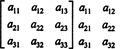Then, multiply down along the three diagonals that start with the first row of the original matrix, and multiply up along the three diagonals tha start with the bottom row of the original matrix. Keep the signs of the three “down” products, reverse the signs of the three “up” products, and add all six resulting terms; this gives (**) Note: This method works only for 3 by 3 matrices.Here's a helpful way to interpret definition (*). Note that in each of the products involved in the sumthere are n factors, no two of which come from the same row or column, a consequence of the bijectivity of every permutation. Using the 3 by 3 case above as a specific example, each of the six terms in the sum (**) can be illustrated as follows:These six products account for all possible ways of choosing three entries, no two of which reside in the same row or column. In general, then, the determinant is the sum of all possible products of n factors, no two of which come from the same row or column of the matrix, with the sign of each product, a 1 j1 a 2 j2a n jn, determined by the sign of the corresponding permutation σ:(1, 2, …, n) ↦( j 1, j 2),…. j n.

Method 2 for defining the determinant. The second definition for the determinant follows from stating certain properties that the determinant function is to satisfy, which, it turns out, uniquely define the function. These properties will then lead to an efficient method for actually computing the determinant of a given matrix.

There exists a unique real‐valued function—the determinant function (denoted det)—which is defined for n by n matrices and satisfies the following three properties:

Property 1: The determinant of a matrix is linear in each row.

Property 2: The determinant reverses sign if two rows are interchanged.

Property 3: The determinant of the identity matrix is equal to 1.

Property 1 deserves some explanation. Linearity of a function f means that f( x + y) = f( x) + f( y) and, for any scalar k, f( kx). Linearity of the determinant function in each row means, for example, thatand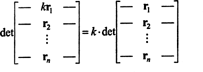Although these two equations illustrate linearity in the first row, linearity of the determinant function can be applied to any row.

Property 2 can be used to derive another important property of the determinant function:

Property 4: The determinant of a matrix with two identical rows is equal to 0.

The proof of this fact is easy: Assume that for the matrix A, Row i = Row j. By interchanging these two rows, the determinant changes sign (by Property 2). However, since these two rows are the same, interchanging them obviously leaves the matrix and, therefore, the determinant unchanged. Since 0 is the only number which equals its own opposite, det A = 0.

One of the most important matrix operations is adding a multiple of one row to another row. How the determinant reacts to this operation is a key property in evaluating it:

Property 5: Adding a multiple of one row to another row leaves the determinant unchanged.

The idea of the general proof will be illustrated by the following specific illustration. Suppose the matrix A is 4 by 4, and k times Row 2 is added to Row 3:By linearity applied to the third row,But the second term in this last equation is zero, because the matrix contains two identical rows (Property 4). Therefore,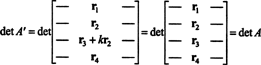The purpose of adding a multiple of one row to another row is to simplify a matrix (when solving a linear system, for example). For a square matrix, the goal of these operations is to reduce the given matrix to an upper triangular one. So the natural question at this point is: What is the determinant of an upper triangular matrix?

Property 6: The determinant of an upper triangular (or diagonal) matrix is equal to the product of the diagonal entries.

To prove this property, assume that the given matrix A has been reduced to upper triangular form by adding multiples of rows to other rows and assume that none of the resulting diagonal entries is equal to 0. (The case of a 0 diagonal entry will be discussed later.) This upper triangular matrix can be transformed into a diagonal one by adding multiples of lower rows to higher ones. At each step of this transformation, the determinant is left unchanged, by Property 5. Therefore, the problem of evaluating the determinant of the original matrix has been reduced to evaluating the determinant of an upper triangular matrix, which in turn has been reduced to evaluating the determinant of a diagonal matrix. By factoring out each diagonal entry and using Property 1 (linearity in each row), Property 3 (det I = 1) gives the desired result: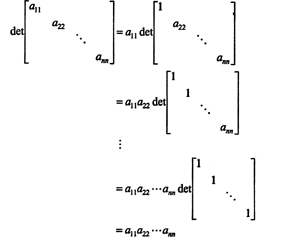Now, to handle the case of a zero diagonal entry, the following property will be established:

Property 7: A matrix with a row of zeros has determinant zero.

This is also easy to prove. As in the proof of Property 5, the essential idea of this proof will also be illustrated by a specific example. Consider the 3 by 3 matrix(Recall that each * indicates an entry whose value is irrelevant to the present discussion.)

Since for any scalar k,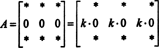linearity of the determinant impliesBut, if det A is equal to k det A for any scalar k, then det A must be 0.

Now, to complete the discussion of Property 6: If a diagonal entry in an upper triangular matrix is equal to 0, then the process of adding a multiple of one row to another can produce a row of zeros. For example,This step does not change the determinant (Property 3), so the determinant of the original matrix is equal to the determinant of a matrix with a row of zeros, which is zero (Property 4). But in this case at least one of the diagonal entries of the upper triangular matrix is 0, so the determinant does indeed equal the product of the diagonal entries. Generalizing these arguments fully establishes Property 6.

Example 6: Evaluate the determinant ofReduce the matrix to an upper triangular one,in order to exploit Property 6—that none of these operations changes the determinant—and Property 7—that the determinant of an upper triangular matrix is equal to the product of the diagonal entries. The result isExample 7: Evaluate the determinant ofThe following elementary row operations reduce A to an upper triangular matrix: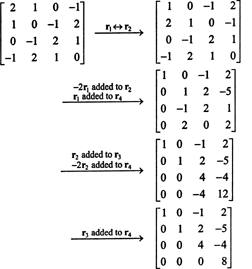None of these operations alters the determinant, except for the row exchange in the first step, which reverses its sign. Since the determinant of the final upper triangular matrix is (1)(1)(4)(8) = 32, the determinant of the original matrix A is −32.

Example 8: Let C be a square matrix. What does the rank of C say about its determinant?

Let C be n x n and first assume that the rank of C is less than n. This means that if C is reduced to echelon form by a sequence of elementary row operations, at least one row of zeros appears at the bottom of the reduced matrix. But a square matrix with a row of zeros has determinant zero. Since no elementary row operation can turn a nonzero‐determinant matrix into a zero‐determinant one, the original matrix C had to have determinant zero also.

On the other hand, if rank C = n, then all the rows are independent, and the echelon form of C will be upper triangular with no zeros on the diagonal. Thus, the determinant of the reduced matrix is nonzero. Since no elementary row operation can transform a zero‐determinant matrix into a nonzero‐determinant one, the original matrix C had to have a nonzero determinant. To summarize then,Example 9: Evaluate the determinant of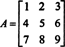None of the following row operations affects the determinant of A: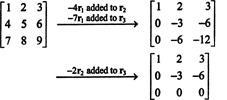Because this final matrix has a zero row, its determinant is zero, which implies det A = 0.

Example 10: What is the rank of the following matrix?Since the third row is a linear combination, r 3 = − r 1 + 2 r 2, of the first two rows, a row of zeros results when A is reduced to echelon form, as in Example 9 above. Since just 2 nonzero rows remain, rank A = 2.

The three preceding examples illustrate the following important theorem:

Theorem E. Consider a collection { v 1, v 2,…, v n } of n vectors from R n . Then this collection is linearly independent if and only if the determinant of the matrix whose rows are v 1, v 2,…, v n is not zero.

In fact, Theorem E can be amended: If a collection of n vectors from R n is linearly independent, then it also spans R n (and conversely); therefore, the collection is a basis for R n .

Example 11: Let A be a real 5 by 5 matrix such that the sum of the entries in each row is zero. What can you say about the determinant of A?

Solution 1. The equation x 1 + x 2 + x 3 + x 4 + x 5 = 0 describes a 4‐dimensional subspace of R 5, since every point in this subspace has the formwhich contains 4 independent parameters. Since every row of the matrix A has this form, A contains 5 vectors all lying in a 4‐dimensional subspace. Since such a space can contain at most 4 linearly independent vectors, the 5 row vectors of A must be dependent. Thus, det A = 0.

Solution 2. If x 0 is the column vector (1, 1, 1, 1, 1) T, then the product A x 0 equals the zero vector. Since the homogeneous system A x = 0 has a nontrivial solution, A must have determinant zero (Theorem G, page 239).

Example 12: Do the matrices in M 2x2 ( R) with determinant 1 form a subspace of M 2x2 ( R)?

No. The determinant function is incompatible with the usual vector space operations: The set of 2 x 2 matrices with determinant 1 is not closed under addition or scalar multiplication, and, therefore, cannot form a subspace of M 2x2 ( R). A counterexample to closure under addition is provided by the matrices I and − I; although each has determinant 1, their sum, I + (− I) = 0, clearly does not.

Example 13: Given that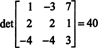(see Example 6), compute the determinant of the matrix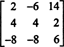obtained by multiplying every entry of the first matrix by 2.

This question is asking for det(2 A) in terms of det A. If just one row of A were multiplied by 2, the determinant would be multiplied by 2, by Property 1 above. But, in this case, all three rows have been multiplied by 2, so the determinant is multiplied by three factors of 2:This gives det(2 A) = 8·40 = 320. In general, if A is an n by n matrix and k is a scalar, then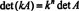Example 14: If A and B are square matrices of the same size, is the equation det ( A + B) = det A + det B always true?

Let A and B be the following 2 by 2 matrices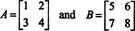Then det A = det B = −2, but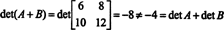Thus, det ( A + B) = det A + det B is not an identity. [Note: This does not mean that this equation never holds. It certainly is an identity for 1 x 1 matrices, and, making just one change in the entries of the matrices above (namely, changing the entry b 22 from 8 to 12),yields a pair of matrices that does satisfy det ( A + B) = det A + det B, as you may check.]

Example 15: One of the most important properties of the determinant function is that the determinant of the product of two square matrices (of the same size) is equal to the product of the individual determinants. That is,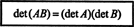is an identity for all matrices A and B for which both sides are defined.

Verify this identity for the matrices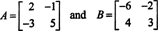Assuming that A is an invertible matrix, what is the relationship between the determinant of A and the determinant of A −1?

If A is a square matrix and k is an integer greater than 1, what relationship exists between det ( A k ) and det A?

The solutions are as follows:

It is easy to see that det A = 7 and det B = −10. The prodct of A and B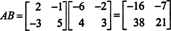has determinant (−16)(21) − (38)(−7) = −336 + 266 = −70. Thus,as expected.

Taking the determinant of both sides of the equation AA −1 = I yields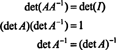Note that the identity (det A)(det A −1) = 1 implies that a necessary condition for A −1 to exist is that det A is nonzero. (In fact, this condition is also sufficient.)

Let k = 2; then det ( A 2) = det ( AA) = (det A)(det A) = (det A) 2. If k = 3, then det ( A 3) = det ( A 2 A) = det ( A 2)(det A) = (det A) 2(det A) = (det A) 3. The pattern is clear: det ( A k ) = (det A) k . [You may find it instructive to give a more rigorous proof of this statement by a straightforward induction argument.]